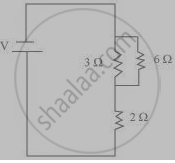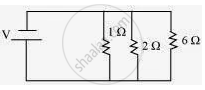Share

# How can three resistors of resistances 2 Ω, 3 Ω and 6 Ω be connected to give a total resistance of (a) 4 Ω, (b) 1 Ω? - CBSE Class 10 - Science

ConceptResistance of a System of Resistors - Resistors in Parallel

#### Question

How can three resistors of resistances 2 Ω, 3 Ω and 6 Ω be connected to give a total resistance of (a) 4 Ω, (b) 1 Ω?

#### Solution

There are three resistors of resistances 2 Ω, 3 Ω, and 6 Ω respectively.

(a) The following circuit diagram shows the connection of the three resistors.H

ere, 6 Ω and 3 Ω resistors are connected in parallel.
Therefore, their equivalent resistance will be given by

1/(1/6+1/3)=(6xx3)/(6+3)=2 Ω

This equivalent resistor of resistance 2 Ω is connected to a 2 Ω resistor in series.
Therefore, the equivalent resistance of the circuit = 2 Ω + 2 Ω = 4 Ω
Hence the total resistance of the circuit is 4 Ω.

(b) The following circuit diagram shows the connection of the three resistors.All the resistors are connected in series. Therefore, their equivalent resistance will be given as

1/(1/2+1/3+1/6)=1/((3+2+1)/6)=6/6=1Omega

Is there an error in this question or solution?

#### Video TutorialsVIEW ALL 

Solution How can three resistors of resistances 2 Ω, 3 Ω and 6 Ω be connected to give a total resistance of (a) 4 Ω, (b) 1 Ω? Concept: Resistance of a System of Resistors - Resistors in Parallel.
S